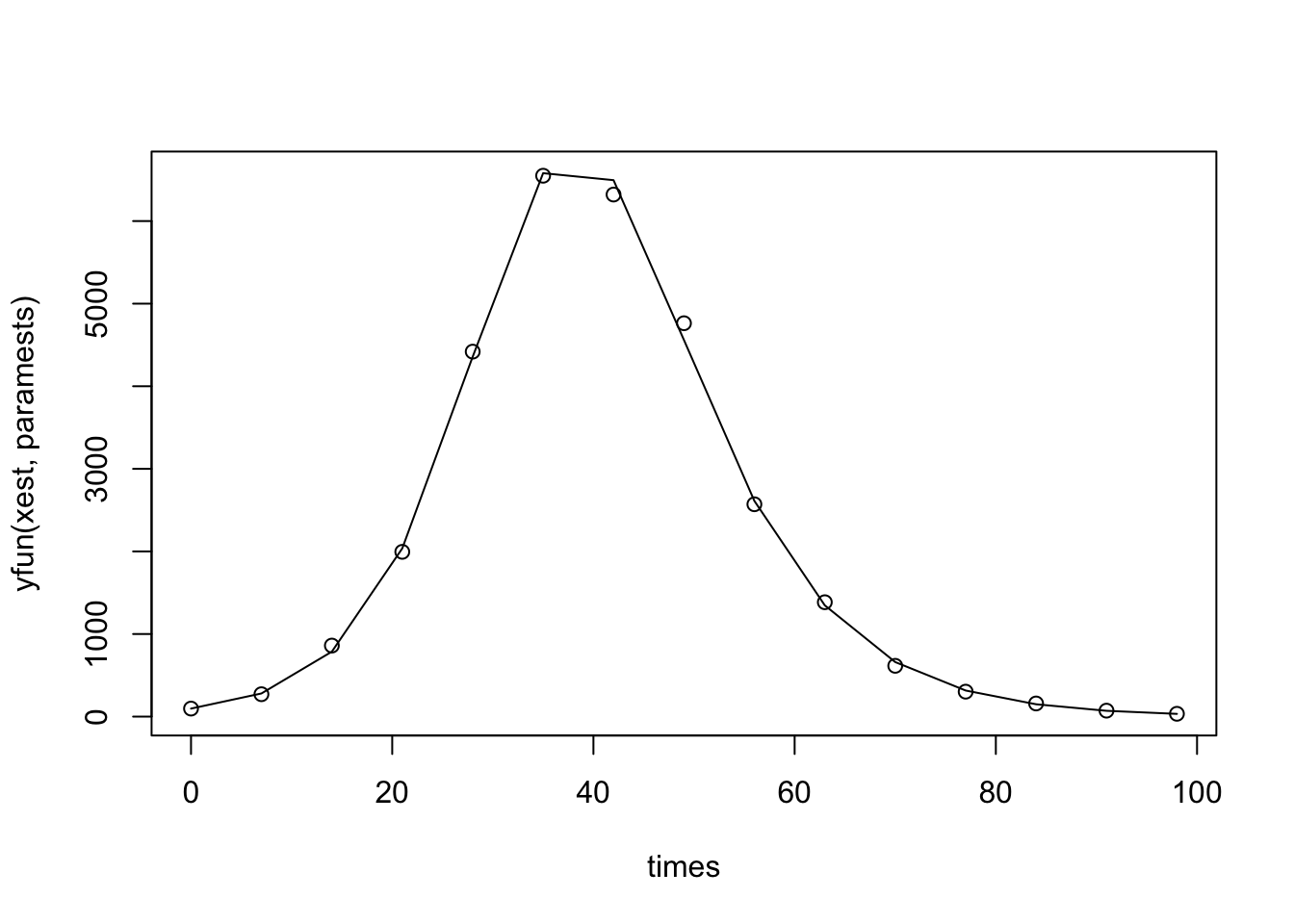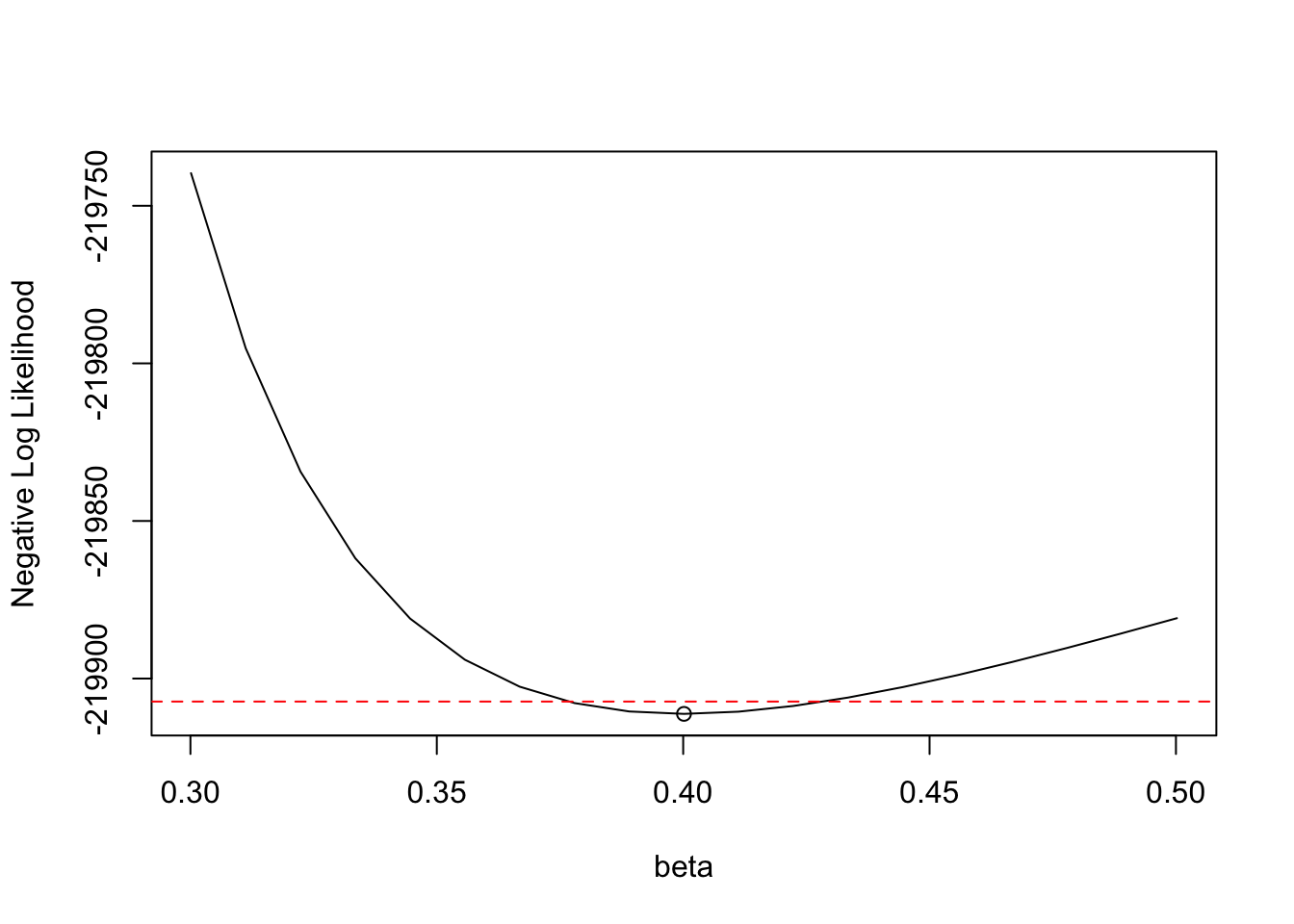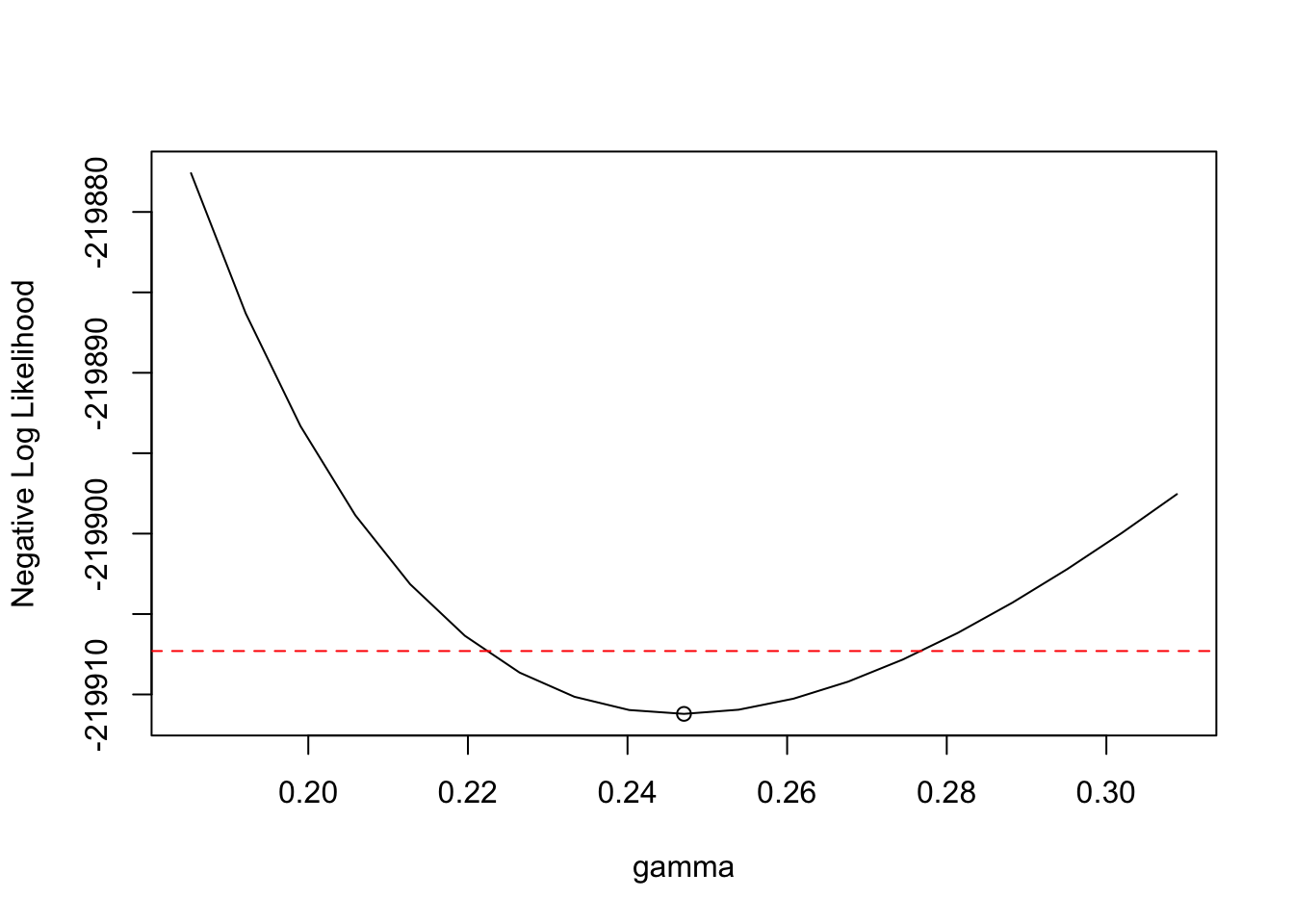## Setup

Start by loading the relevant libraries. We will also be using two extra files (MiniFisher and ProfLike) that you can find on the Epid 814 Github Repository.

library(deSolve)
library(plotly)
library(Matrix)
source('MiniFisher.R')
source('ProfLike.R')

## 1) Structural identifiability for an SIR model

We will consider a version of the classical SIR model that you’ve seen in some of the previous lectures: \begin{aligned} \dot{S} &= \mu N -b S I - \mu S\\ \dot{I} &=b S I-(\mu+\gamma) I\\ \dot{R} &= \gamma I - \mu R\\ \end{aligned} with measurement equation $$y=kI$$. The variables $$S$$, $$I$$, and $$R$$ represent the number of susceptible, infectious, and recovered individuals, and we take $$y$$ to indicate that we are measuring a proportion of the infected population (e.g. if not all cases are reported). The parameters $$\mu, b, \gamma, N$$, and $$k$$ represent (respectively) the birth/death rate, transmission parameter, recovery rate, total population size, and the proportion of the infected population which is reported/observed.

Enter the model into the web app COMBOS, and examine its identifiability. When you enter it into COMBOS, you have to name your state variables using $$x$$’s, so let $$x_1 = S$$ and $$x_2 = I$$.

• Are all the parameters for this model structurally identifiable?

• If any are not, what are the identifiable combinations? Why do you think the combinations have this structure?

• What happens if we re-scale the model to be in terms of fractions of the population instead of individuals? In other words, rescale the model to let $$s = S/N, i = I/N,$$ and $$r = R/N$$. When you do, you will be able to combine some parameters to let $$\beta = bN$$ and $$\kappa = kN$$. Rewrite your model equations in this rescaled and reduced parameter form, and re-run it in COMBOS—how does the identifiability look now?

## 2) Revisiting parameter estimation with the SIR model

We’re going to use the same SIR model and data as we used in the parameter estimation lab. Go there and set up your data, SIR model, initial conditions, measurement equation (yfun), and likelihood function. In case you didn’t set up the SIR model last time, here it is:

SIRode = function(t, x, params){
S = x
I = x
R = x

b = params
g = params

dS = -b*S*I
dI = b*S*I - g*I
dR = g*I

list(c(dS, dI, dR))
}

Estimate the parameters again, and plot your model fit to the data—you should get parameter estimates and a plot something like this (in this case using Poisson maximum likelihood, but you can try other likelihood structures if you’d rather!):

print(paramests)
##         beta        gamma     kappainv
## 4.001581e-01 2.470725e-01 1.243816e-05
plot(times, yfun(xest,paramests), type='l')
points(dataset)## 3) Evaluating identifiability using the FIM

Next, we’ll use the rank of the Fisher information matrix (FIM) to evaluate the identifiability of the model. The function MiniFisher in MiniFisher.R will generate the simplified form of the FIM, $$X^T X$$, which we often use to evaluate identifiability (where $$X$$ is your output sensitivity matrix). We can calculate the FIM as follows:

# Calculate the FIM
FIM = MiniFisher(times,paramests,x0fun2,SIRode,yfun,cases)

Now, we can calulate the rank:

# Calculate the rank
# qr(FIM)$rank rankMatrix(FIM) ##  3 What does this tell us about the identifiability of the model? Try it out with the other un-scaled SIR model given in Part 1) above (or you can use the un-scaled SIR from the parameter estimation lab)—how does that change things? Note: You can use MiniFisher for this problem, but I strongly recommend at least looking at the code in MiniFisher, or better yet, re-coding it yourself to really see how one would calculate a sensitivity matrix, etc. Also, MiniFisher uses a numerical approximation of the derivative—Ariel Cintron Arias’ slides here give a nice introduction to estimation and sensitivity equations using the forward sensitivity equations instead. ## 4) Profile-likelihood-based confidence intervals Next, we’ll calculate profile likelihoods of the parameters and evaluate their identifiability and confidence bounds. Generate profile likelihoods for each of your model parameters ($$\beta, \gamma$$, and $$\kappa$$. You can play with the range to profile the parameters over, but something like $$\pm25\%$$ will likely work well. For the threshold to use in determining your confidence intervals, we note that $$2 (NLL(p) - NLL(\hat{p}) )$$ (where $$NLL$$ is the negative log likelihood) is approximately $$\chi^2$$ distributed with degrees of freedom equal to the number of parameters fitted (including the profiled parameter). Then an approximate 95% (for example) confidence interval for $$p$$ can be made by taking all values of $$p$$ that lie within the 95th percentile range of the $$\chi^2$$ distribution for the given degrees of freedom. In this case, for a 95% confidence interval, we have three total parameters we are estimating ($$\beta, \gamma$$, and $$\kappa$$), so the $$\chi^2$$ value for the 95th percentile is 7.8147. Then the confidence interval is any $$p$$ such that: $NLL(p) \leq NLL(\hat{p}) + 7.8147/2$ In other words, our threshold is $$NLL(\hat{p}) + 7.8147/2 = NLL(\hat{p}) + 3.9074$$, where $$NLL(\hat{p})$$ is the cost function value at our parameter estimates from 2). We can set the confidence interval threshold and range for the profiles like this: threshold = qchisq(0.95,length(paramests))/2 + res$value #threshold for confidence intervals
perrange = 0.25 #percent range for profile to run across

Now let’s generate and plot the profiles. Are your parameters practically identifiable? What are the 95% confidence intervals for your parameters?

profiles = list()
for (i in 1:length(paramests)){
#generate profile
profiles[[i]] = ProfLike(paramests,i,SIRML,times,cases,perrange=perrange)

#plot profile
plot(profiles[[i]]$profparvals, profiles[[i]]$fnvals, type='l',
xlab=names(params)[i], ylab="Negative Log Likelihood",
ylim=c(min(profiles[[i]]$fnvals),max(c(profiles[[i]]$fnvals,threshold))))
points(paramests[i], res$value) abline(h=threshold, col="red", lty="dashed") #plot parameter relationships # matplot(profiles[[i]]$profparvals, profiles[[i]]\$paramestvals, type='l',xlab=names(params)[i],ylab="Estimated Parameter Value")
# points(paramests[i], paramests)
# legend("topleft",inset=.05,names(params),col=1:length(params),cex=0.8,fill=seq_len(length(params)))
}
##  "Starting profile..."
##  1 1
##  1 2
##  1 3
##  1 4
##  1 5
##  1 6
##  1 7
##  1 8
##  1 9
##   1 10
##  2 1
##  2 2
##  2 3
##  2 4
##  2 5
##  2 6
##  2 7
##  2 8
##  2 9
##   2 10##  "Starting profile..."
##  1 1
##  1 2
##  1 3
##  1 4
##  1 5
##  1 6
##  1 7
##  1 8
##  1 9
##   1 10
##  2 1
##  2 2
##  2 3
##  2 4
##  2 5
##  2 6
##  2 7
##  2 8
##  2 9
##   2 10##  "Starting profile..."
##  1 1
##  1 2
##  1 3
##  1 4
##  1 5
##  1 6
##  1 7
##  1 8
##  1 9
##   1 10
##  2 1
##  2 2
##  2 3
##  2 4
##  2 5
##  2 6
##  2 7
##  2 8
##  2 9
##   2 10